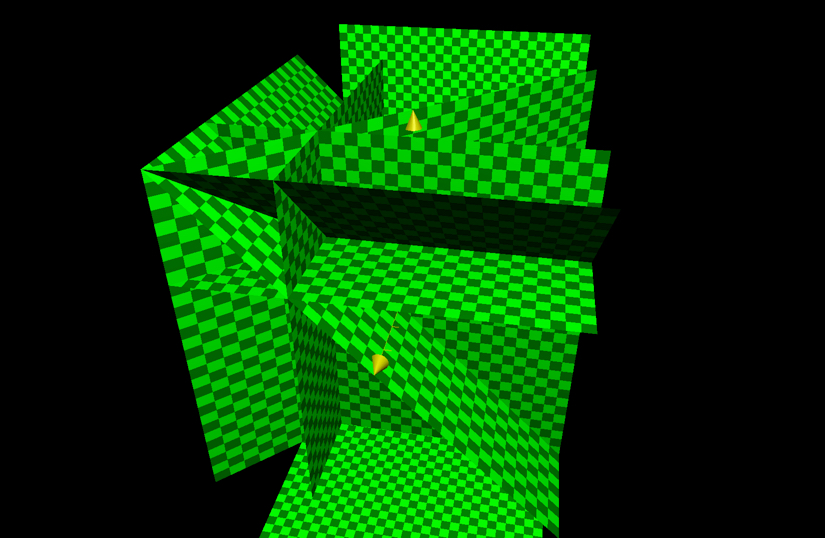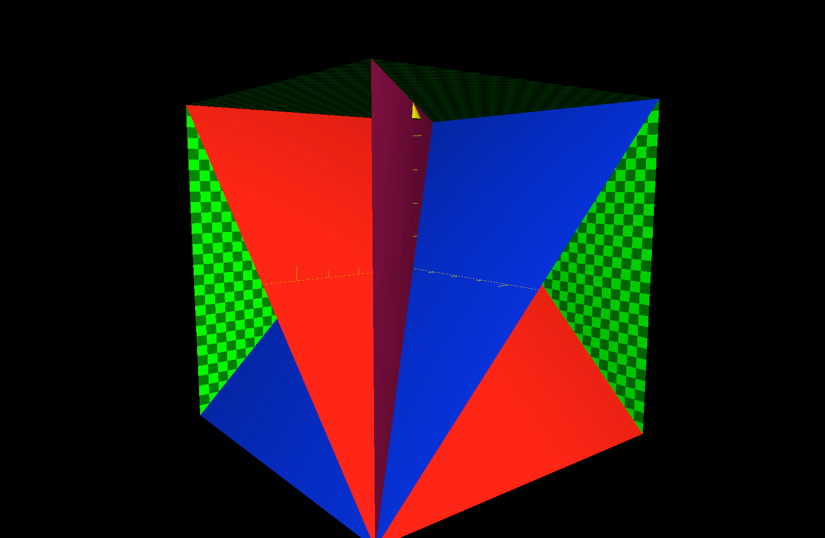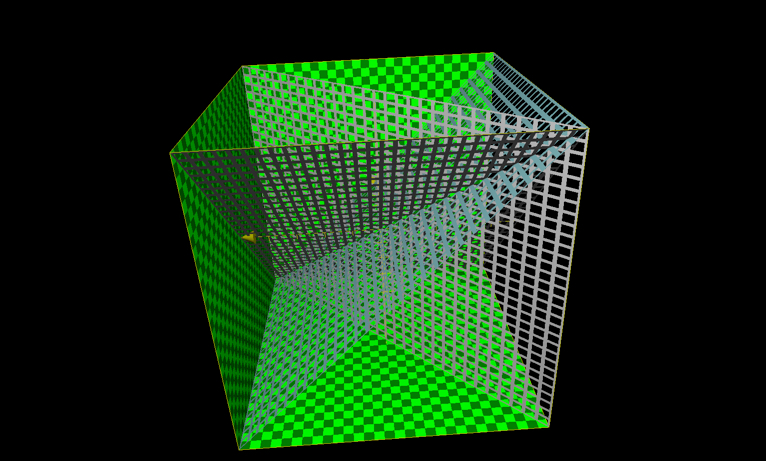Examining the birthday paradox but with a geometric lens.

read moreIf we permute two people’s possible birthdays we get a total of 365 squared possibilities. Now, we can imagine this visually (and represent it geometrically) with two lines each of which belong to each person with 365 units or configurations. When we imagine the possibilities in this setting we imagine a plane, the points lying within representing a single combination of the two birthdays (ex: 5,35 (the 5th day and the 35th day). We can see that the number of days when the two people’s birthdays are the same is the line person1=person2, or on a Cartesian coordinate plane: x=y. This leads to an interesting property of permutation space: the diagonal (the line x=y) in this case is equal to 365 as opposed to the square root of (2*365^2).

The same can be done with three people. We add a third axis (person3 or z) and we get a cube of possibilities. This time, though, we find that we must have the lines: x=y; x=z, and y=z.We end up getting 3*365^2 - 2*365. The first term counts the line of intersection (the length of which is 365) between the three planes three times which means we have to compensate by subtracting 2*365 so that the line of intersection is only counted once.

This can be easily visualized and graphed.The same cannot be said for a four person scenario; it is much more complex. We must draw out the aforementioned cube of possible combinations with another axis: w. We easily represent the number of combinations with: 365^4. It’s amazing that such simple notation can represent something so difficult for humans to visualize. We find that the following cubes of intersection (birthday on the same day) exist:

x=y
x=z
y=z
w=x
w=y
w=z

The last three are the newly added conditions. As we can only perceive three geometric dimensions, this poses a problem.

We know that x=y , x=z, and y=z are invariant with respect to the w-axis. i.e. if we imagine a cube at w=2, for instance, we see that the planes x=y , x=z, and y=z are in the exact same place as at w=1. We are forced to imagine a cube that corresponds to a single value of w.

The last three conditions (w=x,w=y, and w=z) are w-variant. This means that if we vary w, we will see a change in the location of these axes. This makes the calculation of the intersection between w conditions and non-w conditions remarkably difficult. Within w and non-w conditions, the intersection is a lot simpler.

I’m taking the approach of looking at the cube at one value of w and looking at the intersection between a w=? plane and the non-w planes one at a time (first look at the intersection of w=z with x=y, x=z, and y=z, then add the w=y). Once I find the intersections for one cube I’ll make sure they work for all cubes (all values of w) and then I’ll multiply by 365.

We have 6 planes each of which have an area of 365^2. So, without accounting for double counts (the days where person x=y&&w=y), we would have a total overlap (for any given w) of 6*365. This is not the case however because there are some points that are double counted (corresponding to intersections of the lines). Therefore, we must account for this by subtracting from this value.

We start by simply subtracting 2*365 as the planes x=y , x=z, and y=z intersect at only one line (x=y&&y=z), which means that we are counting that line as meeting the condition that two people have the same birthday three times when we should only be counting it once. So we subtract 2*365.

Now, we must think about the next plane introduced: w=z.

At any given w, we have w=z intersecting each of the x=y, x=z, and y=z axes along one line. So, we must do as we did before: subtract for each overlap. Because the line w=z intersects at one line for each of the three planes, we must subtract 3*365. BUT, we see that, when subtracting this value, we are counting one point (where x=y&&y=z&&x=w, i.e. all people have the same birthday) three times when we should only be counting it once. So, we must ADD WHERE INTERSECTIONS MEET INTERSECTIONS to compensate for this over-subtraction. So, we add 2 (because we subtract a singular point two too many times). Thus, for the planes x=y , x=z, y=z, and w=z, we get:

6*365^2 - 2*365 - 3*365 +2. It turns out that the other planes behave the same way so we would the identical equation so we have:

Keep in mind that this is only for one value of w, not for all of them. Fortunately, as w varies, the number of intersections remain the same. That means that we may multiply by 365 to get the total number.

So, for all the combinations of birthdays for four people, the number of days on which at least two people have the same birthday is:

`365 * (6 * 365^2 - 11 * 365 + 6)` or `6 * 365^3 - 11 * 365^2 + 6 * 365`

Found rule: subtract any overlap, add overlap of overlap,